# Ideals and Divisors

Suppose that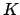is a finite extension of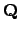. Let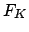be the the free abelian group on a set of symbols in bijection with the non-archimedean valuation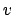of. Thus an element ofis a formal linear combination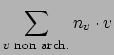where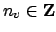and all but finitely many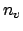are 0.

Lemma 21.2.1   There is a natural bijection betweenand the group of nonzero fractional ideals of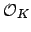. The correspondence is induced by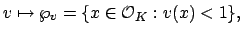whereis a non-archimedean valuation.

Endowwith the discrete topology. Then there is a natural continuous map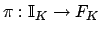given by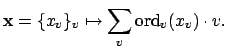This map is continuous since the inverse image of a valuation(a point) is the product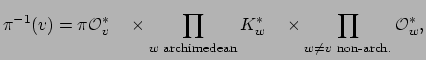which is an open set in the restricted product topology on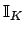. Moreover, the image of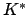inis the group of nonzero principal fractional ideals.

Recall that the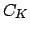of the number fieldis by definition the quotient ofby the image of.

Theorem 21.2.2   The class groupof a number fieldis finite.

Proof. We first prove that the map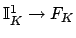is surjective. Let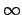be an archimedean valuation on. Ifis a non-archimedean valuation, let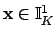be a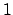-idele such that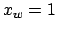at ever valuation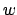exceptand. At, choose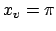to be a generator for the maximal ideal of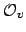, and choose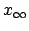to be such that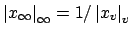. Then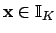and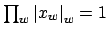, so. Also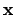maps to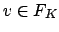.

Thus the group of ideal classes is the continuous image of the compact group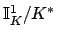(see Theorem 21.1.12), hence compact. But a compact discrete group is finite.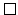Subsections
William Stein 2004-05-06